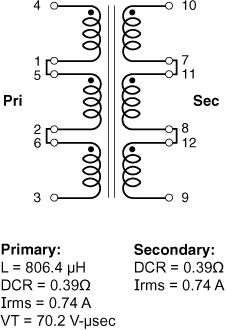## Website-Einstellungen

##### Währungseinstellungen
Produkte
HF
EMI
Informationsmaterial
Produktdokumentation
Handhabung und Verarbeitung
Modelle und Layout-Tools
Kurse/Schulung
Allgemein
Video-Bibliothek
Cx Family Common Mode Chokes
0402CT Low Profile Chip Inductors
XAL7050 High-inductance Shielded Power Inductors
XGL4020 Ultra-low DCR Power Inductors
Quality
Qualitätszertifizierung
Materialzertifizierung
Sicherheitszertifizierung
Zuverlässigkeit
Handhabung / Verarbeitung
Über
Über
Jobs
Standorte
Informationsmaterial

# Application example

for Hexa-Path – Gate driver

<- Back

## Formulas used to calculate electrical characteristics

Connecting windings in series:

• Inductance = Inductance(table) × (number of windings)2
• DCR = DCR(table) × number of windings
• Isat = (Isat(table) × 6) ÷ number of windings connected in series
• Irms = Irms(table)

Connecting windings in parallel:

• Inductance = Inductance(table)
• DCR = 1 ÷ [number of windings × (1 ÷ DCR(table))]
• Isat = (Isat(table) × 6) ÷ number of windings connected in series
• Irms = Irms(table) × number of windings

### Create a 1:1 gate drive transformer

Choose HP1-1400L

Part number Inductance (µH) Percent Tolerance DCR max (Ω) Volt-time product (V-µs) Irms (A)
HP1-1400L 89.6 25 0.13 23.4 0.74

Connecting primary windings in parallel:
When primary windings (Wpri) are connected in series, inductance and volt-time product increase, energy storage and Irms remain the same, but DCR increases.

Example: For HPH1-1400L, connect three primary windings in series:
• Inductance = Inductance(table) × Wpri2 = 89.6 × 9 = 806.4 μH
• DCR = DCR(table) × Wpri = 0.130 × 3 = 0.39 Ohms
• VT = VT(table) × Wpri = 70.2 V-μsec
• Irms = Irms(table) = 0.74 AConnecting secondary windings in parallel:
When secondary windings (Wsec) are connected in series, Irms
remains the same, but DCR increases.

Example: For HP1-1400L, connect three secondary windings in series:
• DCR = DCR(table) × Wsec = 0.130 × 3 = 0.39 Ohms
• Irms = Irms(table) = 0.74 A